# Black-Scholes formula

(Redirected from Black–Scholes formula)

In 1973, F. Black and M. Scholes published a formula for the price of a financial contract whose pay-off at a future time depends in a non-linear way on the value of a given asset at that time. The Black–Scholes formula has been remarkably successful both in terms of use within the financial industry and as a starting point for further mathematical research. Nowadays (2000), the theory of pricing of so-called derivative contracts and related subjects has grown into a well-developed mathematical discipline. A survey of the area is available, for instance in [a8].

The best-known example of a derivative contract is the European call option, which gives the holder the right, but not the obligation, to acquire a certain asset (the underlying) at a specified future time (the expiration time) for a specified price (the strike price). If the value of the underlying asset at expiration timeis denoted by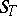and the strike price is denoted by, then the value of the call option at expiration is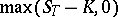. The formula given by Black and Scholes for the value of a European call option at a timeis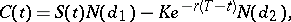(a1)

where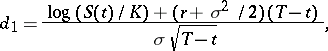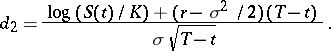The meaning of the symbols is as follows:is the price at timeof a call option expiring at time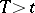with strike price;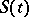is the price of the underlying asset at time;is the cumulative normal distribution function;is the interest rate; andis a parameter known as the volatility of the underlying asset.

The theorem behind the Black–Scholes formula can be stated as follows. Let the real-valued stochastic process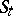and the deterministic function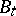satisfy the Itô stochastic differential equations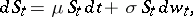(a2)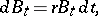where,, andare constants, and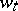denotes a standard Brownian motion (cf. also Stochastic process; Stochastic differential equation). Define a self-financing portfolio strategy as a pair of processesadapted to the filtration associated to the Brownian motion in (a2), such that the process defined by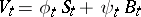(the portfolio value process) satisfies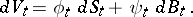(The economic interpretation of this formula is that no funds are added to or withdrawn from the portfolio, whence the terminology "self-financing" .) Given fixed timesandwith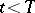and a current asset value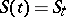, there exists a self-financing strategy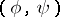defined on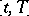such that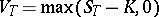almost surely if and only if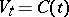, whereis given by (a1).

In the proof of the above theorem, it is shown that the portfolio weight processes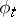and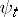can actually be constructed byandwhere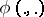and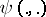are smooth functions. The pair of functionsis called a hedge strategy. To practitioners, the computation of hedge strategies is at least as important as the computation of prices.

The Black–Scholes formula has been extended in many directions. One can consider options that depend on the final valuein other ways, options that depend not just on the final value but also on the path taken by the variable, and options on several underlying variables; moreover, one may consider different specifications of the processes followed by the underlying variables. Options whose time of expiry is fixed are called European options; there are also American options, which expire at a point in time to be selected by the holder. In a number of cases analytical solutions analogous to the Black–Scholes formula can be given, but in many other instances one has to resort to numerical methods. Analytical as well as numerical techniques can be distinguished in two types. One approach is based on the characterization of the option value in terms of the expectation of the value of the option at expiration under a so-called equivalent martingale measure, which is related to the originally given measure through a Girsanov transformation (cf. also Martingale; Controlled stochastic process). The second approach uses a diffusion equation that can be written down for the evolution of the option price as a function of the underlying variables. The two approaches are related via a Feynman–Kac formula.

The idea of using Brownian motion to describe the behaviour of asset prices dates back to the doctoral dissertation of L. Bachelier, which appeared in 1900 [a1]. The model (a2), known as geometric Brownian motion, was proposed by P.A. Samuelson in 1964 [a7]. The original formulation of the Black–Scholes formula can be found in [a2]. Attempts at rigorizing the arguments given in this paper started with [a6]. The interpretation given above in terms of self-financing portfolio strategies is due to J.M. Harrison and D. Kreps [a3] and Harrison and S. Pliska [a4]. Systematic accounts of the theory of option pricing and related issues of financial risk management can be found in several textbooks and at various levels of sophistication; see [a5] for a mathematical treatment.

For additional references, see Option pricing.